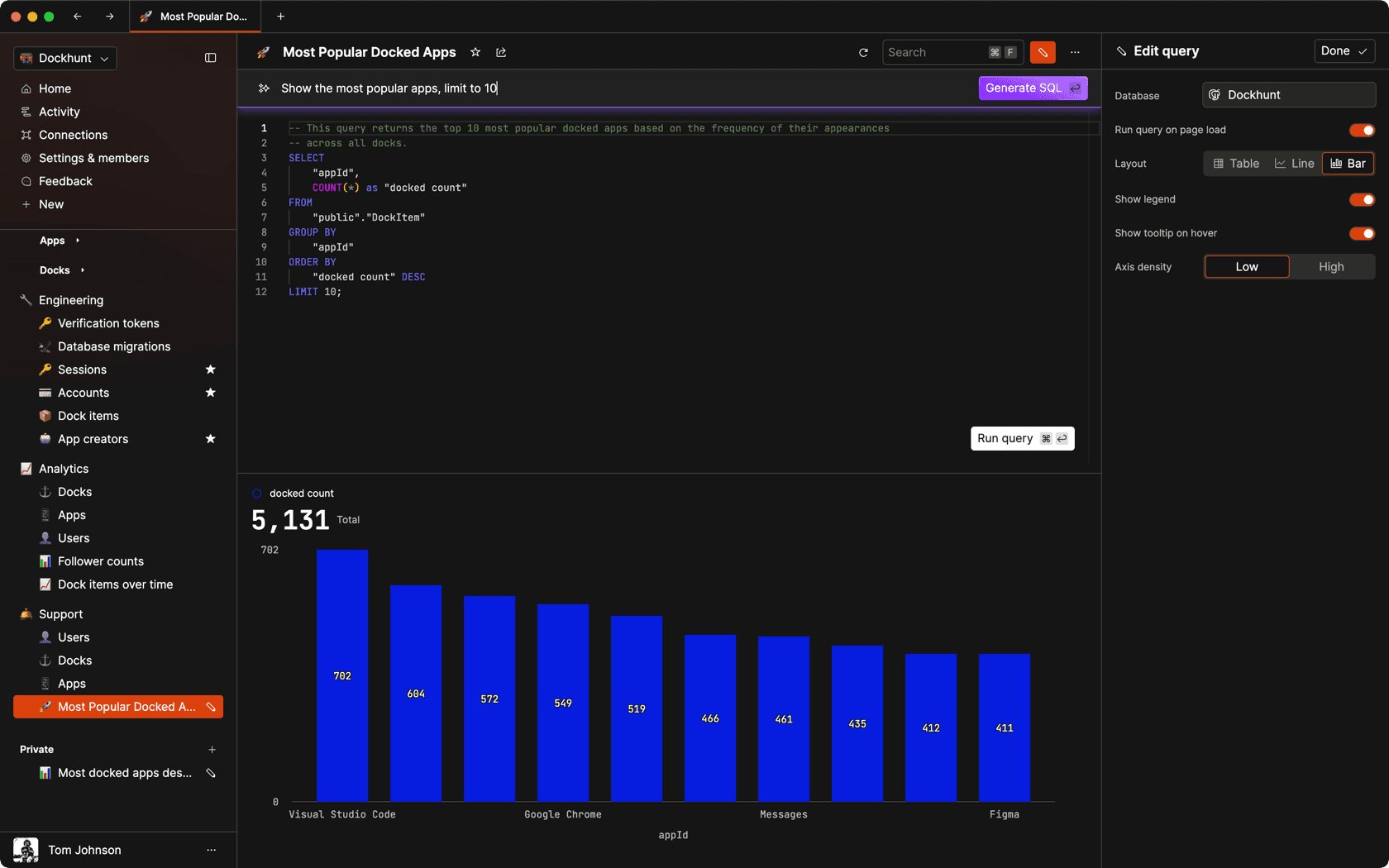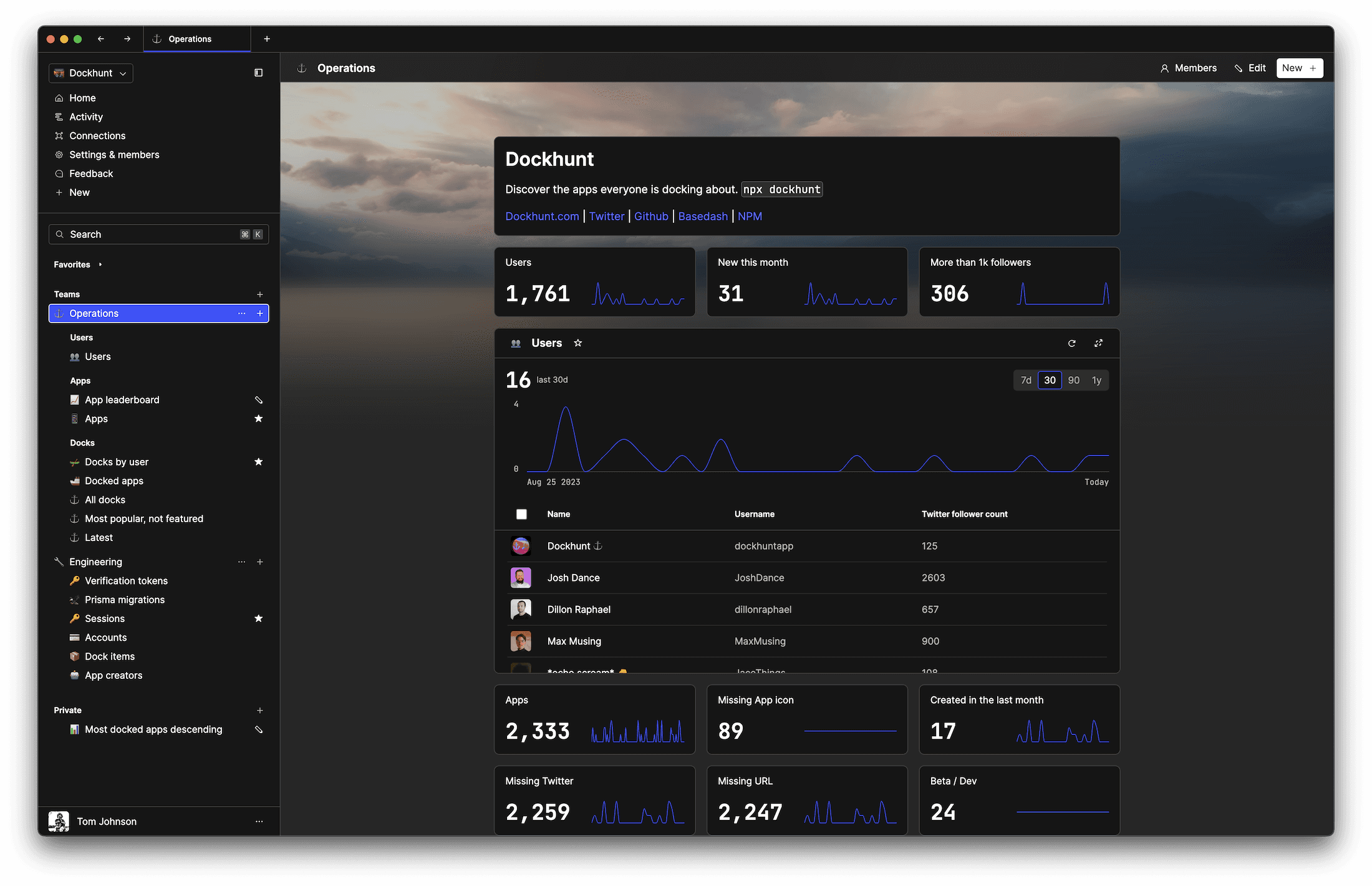# How to generate a random bool in JavaScriptself.__wrap_n=self.__wrap_n||(self.CSS&&CSS.supports("text-wrap","balance")?1:2);self.__wrap_b=(e,r,t)=>{t=t||document.querySelector(`[data-br="\${e}"]`);let n=t.parentElement,a=s=>t.style.maxWidth=s+"px";t.style.maxWidth="";let o,i=n.clientWidth,l=n.clientHeight,c=i/2-.25,f=i+.5;if(i){for(a(c),c=Math.max(t.scrollWidth,c);c+1<f;)o=Math.round((c+f)/2),a(o),n.clientHeight===l?f=o:c=o;a(f*r+i*(1-r))}t.__wrap_o||typeof ResizeObserver<"u"&&(t.__wrap_o=new ResizeObserver(()=>{self.__wrap_b(0,+t.dataset.brr,t)})).observe(n)};self.__wrap_n!=1&&self.__wrap_b(":Rj0pm:",1)

November 6, 2023

You can generate a random boolean in JavaScript using the `Math.random()` function to produce a value that is then evaluated as true or false. We’ll go into more detail on this below.

## Understanding `Math.random()`

JavaScript's `Math.random()` function generates a floating-point, pseudo-random number in the range 0 (inclusive) to 1 (exclusive). This means that every time you call `Math.random()`, you'll receive a new number that could be anything from 0 up to but not including 1.

## Simplest approach: Using `Math.random()`

The most straightforward way to get a random boolean value is by checking if `Math.random()` is greater than 0.5. Since `Math.random()` can return any value between 0 and 1, there is a roughly 50/50 chance that the result will be above or below 0.5.

`const randomBool = Math.random() >= 0.5;`

## Ensuring equal distribution

Although the above method is concise, it may not offer a perfect 50/50 distribution due to the nature of floating-point calculations and the implementation of `Math.random()`. For most purposes, this isn't a concern, but if an exact distribution is critical, you might want to implement additional checks or a different method.

## Using bitwise operators

Bitwise operators can also be used to generate a random boolean. By using the OR operator with zero, you can convert the generated number to an integer, and the double NOT bitwise operator will then convert this integer to a boolean value.

`const randomBool = !!(Math.random() * 2 | 0);`

## Generating multiple random booleans

If you need to generate an array of random booleans, you can use the `Array.from` method in combination with `Math.random()`.

`const randomBools = Array.from({ length: 10 }, () => Math.random() >= 0.5);`

This code snippet creates an array with ten random booleans.

## Using a function for readability

For better readability and reusability, you can wrap the random boolean generation in a function.

```function getRandomBool() {
return Math.random() >= 0.5;
}```

Now, whenever you need a random boolean, you just call `getRandomBool()`.

## Customizing probability

If you want to customize the probability of true or false, you can pass a threshold to the function.

```function getRandomBool(threshold = 0.5) {
return Math.random() < threshold;
}```

By changing the threshold value, you can adjust the probability that true is returned.

## Other ways to generate a random boolean in JavaScript

### The `Boolean` constructor

This method is useful when you want to make the coercion to boolean very explicit in your code, which can enhance readability for those unfamiliar with JavaScript's truthy and falsy values.

`const randomBool = Boolean(Math.floor(Math.random() * 2));`

### Toggling a boolean in a loop

The toggling approach is especially handy in simulations or games where you might want a state to have a chance to change on each iteration, such as flipping a moving character between visible and invisible states to indicate invulnerability.

```let bool = false;
for (let i = 0; i < 10; i++) {
bool = Math.random() >= 0.5 ? !bool : bool;
}```

### Immediately Invoked Function Expression (IIFE)

IIFE is suited for situations where you need an immediate execution and there's no need to reuse the code. It keeps the global scope clean and is useful in modular patterns or self-contained components.

`const randomBool = (() => Math.random() >= 0.5)();`

### The `Date` object

Using the `Date` object for randomness could be applied in scenarios where the randomness is not critical and might be more about adding slight variability to the behavior of a function. For example, alternating styles or animations in a non-security-related context.

`const randomBool = new Date().getMilliseconds() % 2 === 0;`

## Conclusion

While there are several ways to generate a random boolean in JavaScript, the simplest method using `Math.random()` is often sufficient. For more control over the distribution or the probability of true or false, custom functions can be created to suit the specific needs of any project.

# Ship faster, worry less with Basedash

You're busy enough with product work to be weighed down building, maintaining, scoping and developing internal apps and admin panels. Forget all of that, and give your team the admin panel that you don't have to build. Launch in less time than it takes to run a standup.

You're busy enough with product work to be weighed down building, maintaining, scoping and developing internal apps and admin panels. Forget all of that, and give your team the admin panel that you don't have to build. Launch in less time than it takes to run a standup.

You're busy enough with product work to be weighed down building, maintaining, scoping and developing internal apps and admin panels. Forget all of that, and give your team the admin panel that you don't have to build. Launch in less time than it takes to run a standup.### Dashboards and charts

Effortlessly make charts and create a space for your team to work together towards shared goals and metrics.

### SQL composer with AISOC II
Type 2 Certified

### Features

Proudly carbon-neutral.SOC II
Type 2 Certified

### Features

Proudly carbon-neutral.SOC II
Type 2 Certified